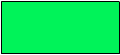# Rotational Symmetry

Rate 0 stars Common Core
Quiz size:
Message preview:
Someone you know has shared Rotational Symmetry quiz with you:

To play this quiz, click on the link below:

https://www.turtlediary.com/quiz/rotational-symmetry.html

Hope you have a good experience with this site and recommend to your friends too.

Login to rate activities and track progress.
Login to rate activities and track progress.

A figure has rotational symmetry if it can be rotated about a point less than a full turn and look the same as it did before the rotation.

The order of rotational symmetry is the number of times a figure looks the same as it did originally when it is rotated through 360°.

For example: Consider this figure.If we rotate it one-half turn, it will look the same.So, it has rotational symmetry.

Also, observe that when the figure is rotated through 360°, it looks the same two times.So, this figure has rotational symmetry of order 2.

Even if a figure has no rotational symmetry, the order of rotational symmetry will still be 1 as every figure looks the same at the end of a complete rotation (360°).

For example: Consider this figure.The order of rotational symmetry of this figure is 1.

ds

A B C D E F G H I J K L M N O P Q R S T U V W X Y Z